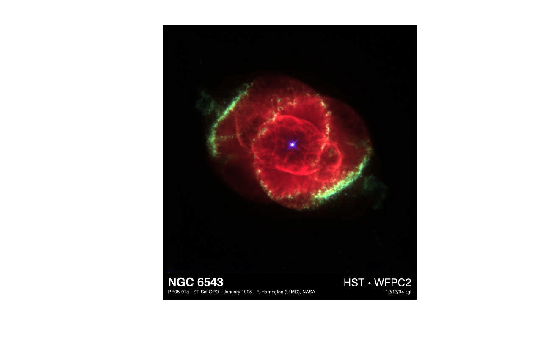## Syntax

``[RGB,alpha] = readRGBAImage(t)``

## Description

example

````[RGB,alpha] = readRGBAImage(t)` reads the image and alpha matting data from the TIFF file associated with the `Tiff` object `t` using the RGBA interface.```

## Examples

collapse all

Get all image data and its associated alpha matting from a TIFF file.

Create a `Tiff` object for an image file.

`t = Tiff('example.tif','r');`

Read the image data and the associated alpha matting.

`[RGB,A] = readRGBAImage(t);`

Display the image.

`imshow(RGB)`Close the `Tiff` object.

`close(t);`

## Input Arguments

collapse all

`Tiff` object representing a TIFF file. Use the `Tiff` function to create the object.

## Output Arguments

collapse all

Image data, returned as a `m`-by-`n`-by-`3` numeric array. Where `m` and `n` are the height and width of the image, respectively.

The `readRGBAImage` function can transform the pixel values based on specifications in these tags:

 `PhotometricInterpretation` `BitsPerSample` `SamplesPerPixel` `Orientation` `ExtraSamples` `ColorMap`

Alpha matting associated with the image, returned as an `m`-by-`n` numeric array. Where `m` and `n` are the height and width of the image, respectively.

If the image does not have associated alpha matting, then `alpha` is a matrix with all values set to `255` (transparent).

## Algorithms

collapse all

### References

This function corresponds to the `TIFFReadRGBAImage` function in the LibTIFF C API. To use this function, you must be familiar with the TIFF specification and technical notes. View this documentation at LibTIFF - TIFF Library and Utilities.

## Version History

Introduced in R2009b